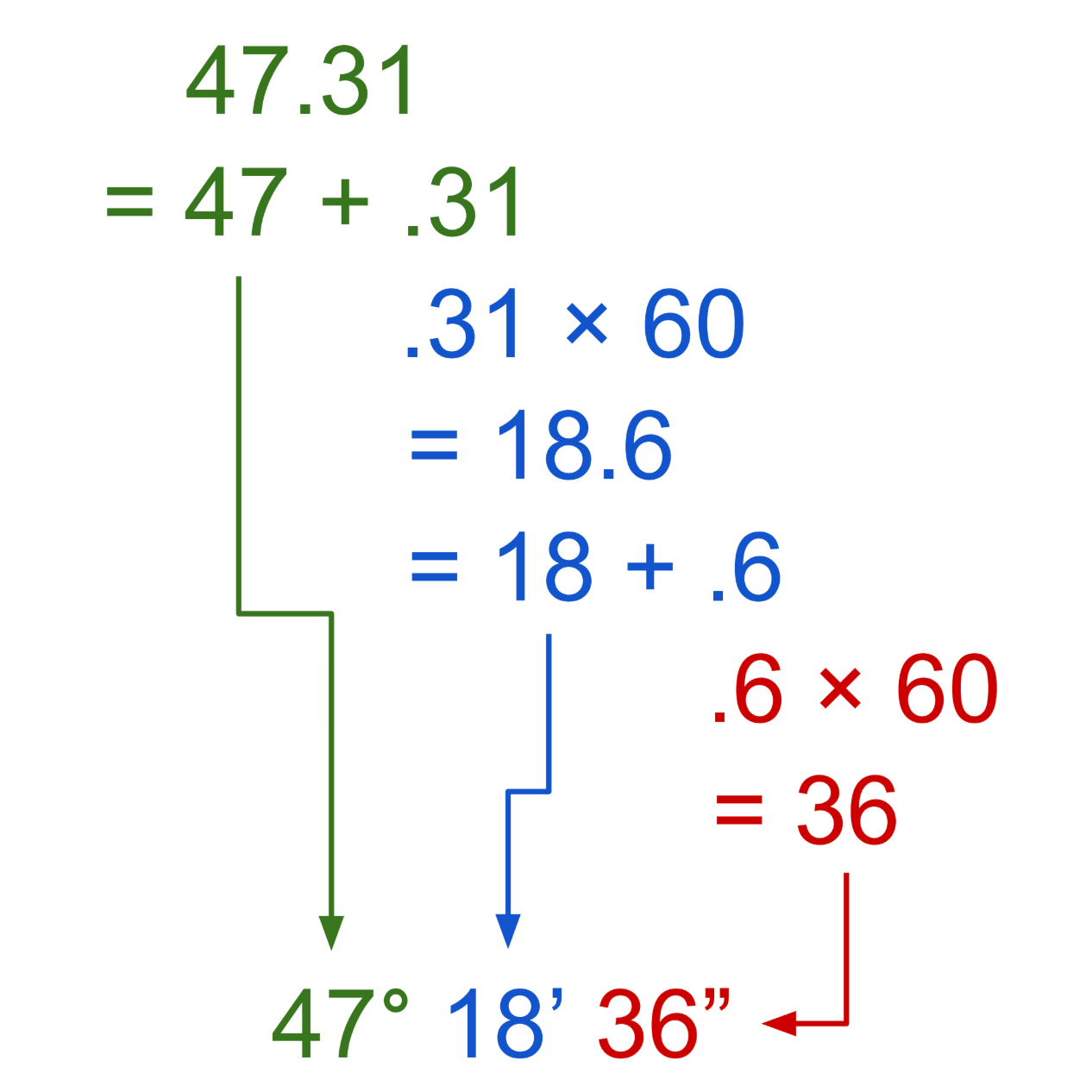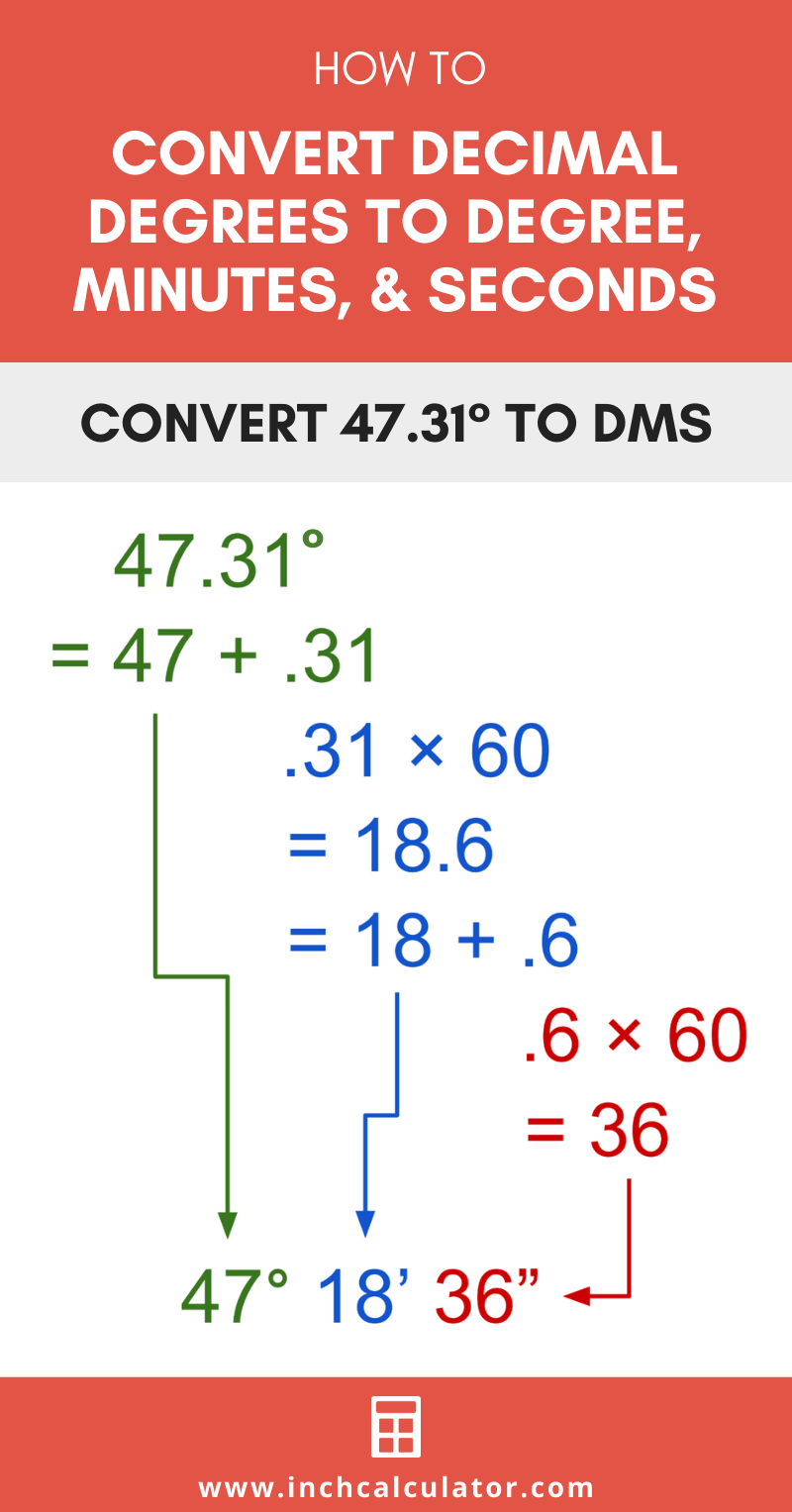# Decimal Degrees to Degrees, Minutes, Seconds Calculator

Enter decimal degrees to convert to minutes and seconds. See the formulas below to see how it’s done.

Angle:
°

## Degrees, Minutes, & Seconds:

47° 18′ 36″
Learn how we calculated this below

Try our calculator to convert degrees, minutes, and seconds to decimal.

## How to Convert Decimal Degrees to Degrees, Minutes, Seconds (DMS)

Degrees are commonly expressed using degrees, minutes, and seconds, otherwise referred to as DMS. In this representation, degrees are the whole units without any decimal places.

Minutes (′) are used to express the part of the angle that is smaller than one degree, and seconds (″) are used to express the part that is smaller than one minute.

There are a few simple steps to convert decimal degrees to degrees with minutes and seconds.

### Step One: Find Whole Degrees

Since minutes and seconds are used to express the measurement of an angle that is smaller than one degree, start by setting the number to the left of the decimal point as the degrees.

### Step Two: Find Minutes

The decimal point, or remainder of the angle that is smaller than one degree can be converted to minutes and seconds. Multiply this decimal value by 60 to find the number of minutes.

minutes = remaining decimal × 60

This resulting number is the minutes and seconds. The whole number to the left of the decimal point is the number of minutes. The remainder is the number of partial minutes, which need to be converted to seconds.

### Step Three: Find Seconds

Multiply the remaining decimal value by 60 again to find the number of seconds.

seconds = remaining decimal × 60

### Step Four: Rewrite Decimal as Degree, Minutes, & Seconds

Finally, express the whole degrees, minutes, and seconds with their respective symbols

D° M′ S″

## Decimal to DMS Example

For example, convert 47.31° to DMS.

Find the whole degrees:
47.31° = 47° + .31°
degrees = 47°

Find minutes:
minutes = .31 × 60 = 18.6′
minutes = 18′ + .6′
minutes = 18′

Find seconds:
seconds = .6′ × 60
seconds = 36″

Thus, 47.31° is equal to 47° 18′ 36″.Here’s a fun fact: there are 360 degrees in a circle, and each degree is composed of 60 minutes or 3,600 seconds. That means that there are 21,600 minutes around a circle and 1,296,000 seconds around a circle.